Sarthak Arora — October 2, 2020

This article was published as a part of the Data Science Blogathon.

## Understanding the problem of Overfitting in Decision Trees and solving it by Minimal Cost-Complexity Pruning using Scikit-Learn in Python

Decision Tree is one of the most intuitive and effective tools present in a Data Scientist’s toolkit. It has an inverted tree-like structure that was once used only in Decision Analysis but is now a brilliant Machine Learning Algorithm as well, especially when we have a Classification problem on our hands.

These decision trees are well-known for their capability to capture the patterns in the data. But, excess of anything is harmful, right? Decision Trees are infamous as they can cling too much to the data they’re trained on.

Hence, our tree gives poor results on deployment because it cannot deal with a new set of values.But, don’t worry! Just like a skilled mechanic has wrenches of all sizes readily available in his toolbox, a skilled Data Scientist also has his set of techniques to deal with any kind of problem. And that’s what we’ll explore in this article.

## The Role of Pruning in Decision Trees

Pruning is one of the techniques that is used to overcome our problem of Overfitting. Pruning, in its literal sense, is a practice which involves the selective removal of certain parts of a tree(or plant), such as branches, buds, or roots, to improve the tree’s structure, and promote healthy growth. This is exactly what Pruning does to our Decision Trees as well. It makes it versatile so that it can adapt if we feed any new kind of data to it, thereby fixing the problem of overfitting.

It reduces the size of a Decision Tree which might slightly increase your training error but drastically decrease your testing error, hence making it more adaptable.

Minimal Cost-Complexity Pruning is one of the types of Pruning of Decision Trees.

This algorithm is parameterized by α(≥0) known as the complexity parameter.

The complexity parameter is used to define the cost-complexity measure, Rα(T) of a given tree T: Rα(T)=R(T)+α|T|

where |T| is the number of terminal nodes in T and R(T) is traditionally defined as the total misclassification rate of the terminal nodes.

In its 0.22 version, Scikit-learn introduced this parameter called ccp_alpha (Yes! It’s short for Cost Complexity Pruning- Alpha) to Decision Trees which can be used to perform the same.

## Building the Decision Tree in Python

We will use the Iris dataset to fit the Decision Tree on. You can download the dataset here.

First, let us import the basic libraries required and the dataset:

The Dataset looks like this: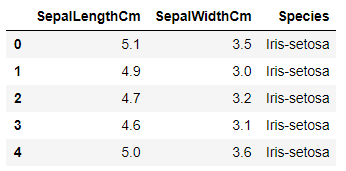Our aim is to predict the Species of a flower based on its Sepal Length and Width.

We will split the dataset into two parts – Train and Test. We’re doing this so that we can see how our model performs on unseen data as well. We shall use the train_test_split function from sklearn.model_selection to split the dataset.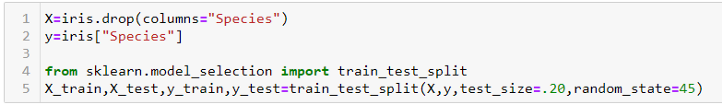Now, let’s fit a Decision Tree to the train part and predict on both test and train. We will use DecisionTreeClassifier from sklearn.tree for this purpose.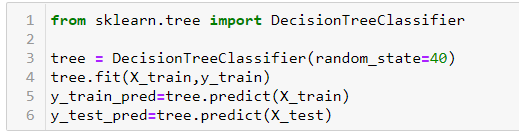By default, the Decision Tree function doesn’t perform any pruning and allows the tree to grow as much as it can. We get an accuracy score of 0.95 and 0.63 on the train and test part respectively as shown below. We can say that our model is Overfitting i.e. memorizing the train part but is not able to perform equally well on the test part.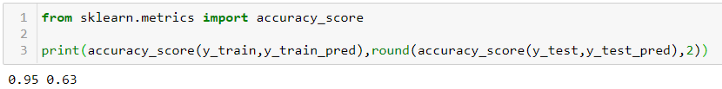DecisionTree in sklearn has a function called cost_complexity_pruning_path, which gives the effective alphas of subtrees during pruning and also the corresponding impurities. In other words, we can use these values of alpha to prune our decision tree: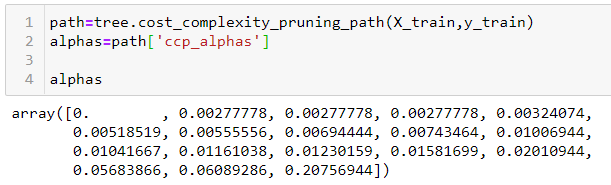We will set these values of alpha and pass it to the ccp_alpha parameter of our DecisionTreeClassifier. By looping over the alphas array, we will find the accuracy on both Train and Test parts of our dataset.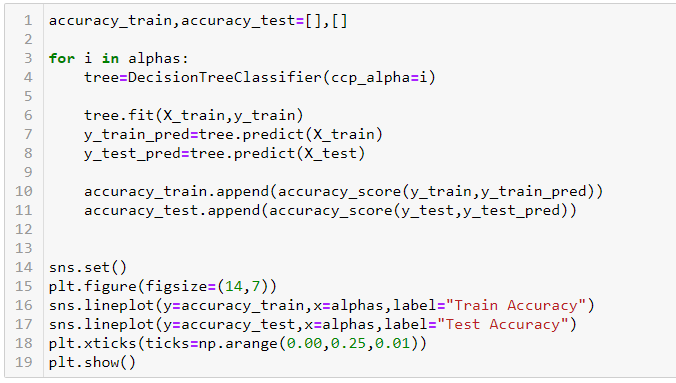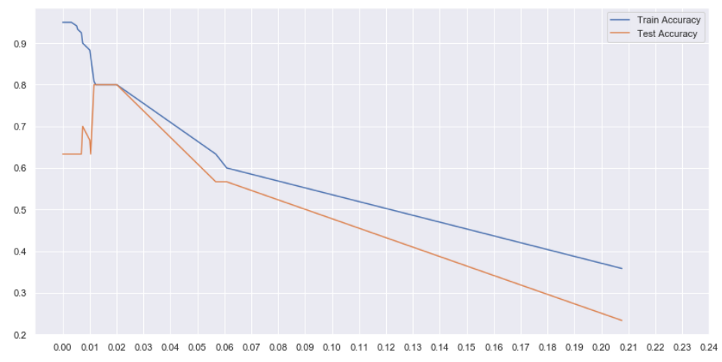From the above plot, we can see that between alpha=0.01 and 0.02, we get the maximum test accuracy. Although our train accuracy has decreased to 0.8, our model is now more generalized and it will perform better on unseen data.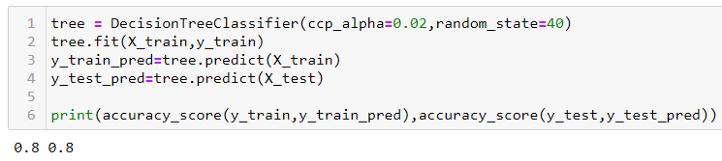## End Notes

If you want to understand the Math behind Cost-Complexity Pruning, click here. Check out the scikit-learn documentation of Decision trees by clicking here.

You can find the notebook on my GitHub and take a closer look at what I have done. Also, connect with me on LinkedIn, and let’s discuss Data!